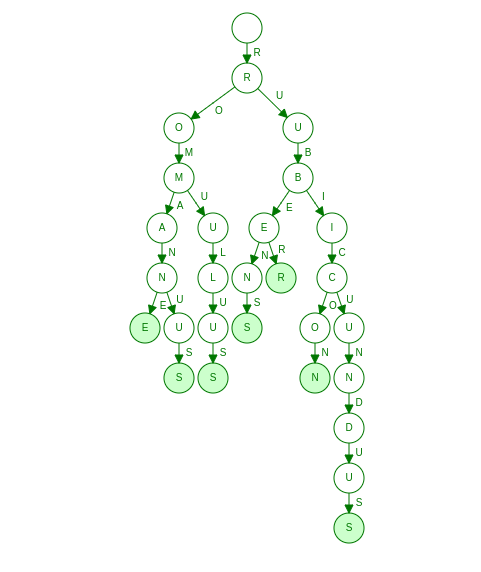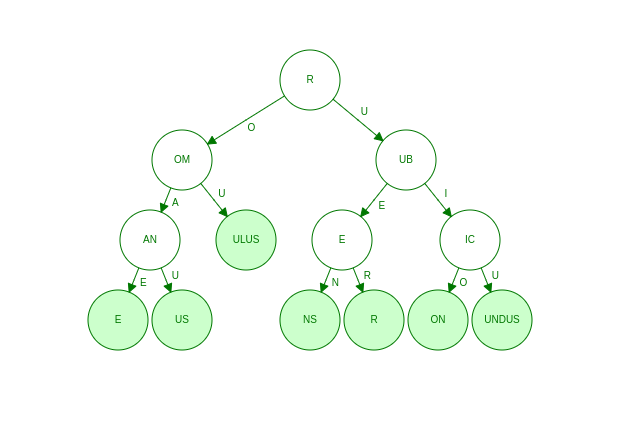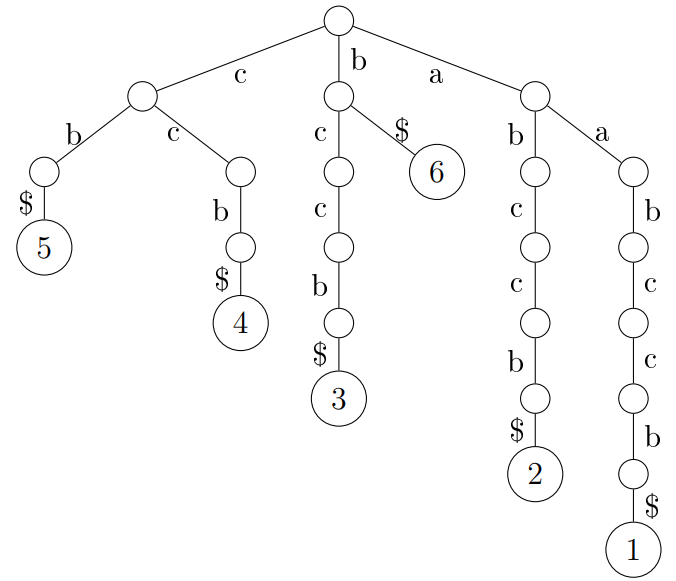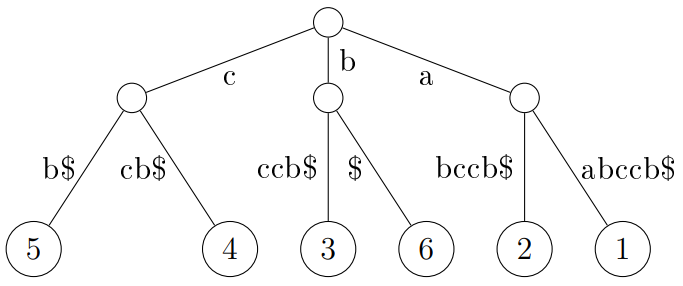# Suffix Tree (A Compressed Version of Trie Data Structure)

In the previous blog, we studied the properties and applications of the trie data structure. In this blog, we will study Suffix trees, an advanced version of tries.

In a trie, each node represents a single element. This representation is not efficient, as we can see in the image below, where the words Romane, Romanus, Romulus, Rubens, Ruber, Rubicon, and Rubicundus are represented in a trie structure.We can see that the letters "O", "A" from the word "ROMANE" and the letters "U", "N", "D", "U", and "S" from the word "RUBICUNDUS" have only one child. If we store these letters together in a single node, we will not lose out on any information.

Although tries have good time complexity, they are space inefficient as they store only one letter in an edge. We remove this space inefficiency by keeping multiple characters of a word in a single node reducing the number of extra edges and nodes needed while maintaining the same symbolic meaning and the performance of tries. In the worst-case scenario, the space required by a compressed trie will be equivalent to that of a simple trie.

The following image shows how the compressed trie will look after inserting all the previously inserted words in the trie.The internal nodes will have at least two children in a compressed trie. Also, it has almost N leaves, where N is the number of strings inserted in the compressed trie. Combining these facts, we can conclude that there are at most 2N -1 nodes in the trie. So the space complexity of a compressed trie is O(N) compared to the O(N²) of a standard trie. Efficient storage is one of the reasons to use compressed tries over classic tries.

Suffix trees are also a compressed version of the trie that includes all of a string's suffixes. Various string-related problems can be solved using suffix trees, which are discussed later in the blog.

Let S be a string of length n. S[i, j] denotes the substring of S from index i to j. Before constructing the suffix tree, we concatenate a new character, \$, to S. By adding this unique character to the end of S, we can avoid cases when a suffix is a prefix of another suffix.

A suffix tree is a rooted, directed tree. It has n leaf nodes labeled from 1 to n, and its edges are labeled by the letters. On a path from the root to the leaf j, one can read the string's suffix S[j, n] and a \$ sign. Such a path is denoted by P(j), and its label is referred to as the path label of j and denoted by L(j).

Image of suffix tree for the word “aabccb”Suffix trees use much space, as we can see from the above image. There are long branches that can be compressed, as discussed earlier. A compact suffix tree of S is a rooted directed tree with n leaves. Each internal node has at least two children. The label of a path is the concatenation of the labels on its edges.

Image of compressed suffix tree for the word “aabccb”### Brute Force algorithm for constructing suffix trees

The brute force algorithm to construct a suffix tree is to consider all suffixes of a string and insert them one by one into a trie. This will take O(N²) time as there are N suffixes, and each suffix will take O(N) time to insert into the trie. This method of constructing suffix trees is inefficient for large strings, and we need to find a better method to construct the suffix tree.

### Ukkonen's algorithm for constructing suffix trees

The basic idea of Ukkonen's Algorithm is to divide the process of constructing a suffix tree into phases. Further, each phase is divided into extensions. In phase i, the ith character is considered. In phase i, all the suffixes of the string S[1:i] are inserted into the trie, and inserting the jth suffix, S[j: i], in a phase is called the jth extension of that phase. So, in the ith phase, there are i extensions and overall there are N such phases. It looks like this is O(N²) task, but the algorithm exploits the fact that these are suffixes of the same string and uses different mechanisms that bring down the time complexity to O(N).

We will now see how an extension step works. We will consider the jth extension of the ith phase. Extending the path S[j:i-1] by adding the letter S[i] is known as the suffix extension. As we are in the ith phase right now, all S[1:i-1] substrings have already been inserted into the tree in the i-1th phase. First, find the last character of S[j:i-1] and then extend it to make sure that S[j:i-1] + S[i](S[j:i]) occurs in the tree. For the extension, the
following rules have to be considered:

1. The complete string S[j:i-1] is found in the tree, and the path ends at a leaf node. In this case, we append the character to the last edge label, and no new nodes are created.
2. If at the end of S[j:i-1] there is no path started with S[i], create a new edge from this position to a new leaf, and label it with S[i]. If this position is inside an edge, then create here a new node and divide the label of the original edge.
3. If S[j: i] is already in the tree, do nothing.

We can see that for each extension, we have to find the path of a string S[j: i] in the tree built in previous phases. The complexity for doing this in the brute force manner will be O(N) for each extension, and the overall time complexity will be O(N³) for building the suffix tree.

Ukkonen's linear-time algorithm is a speed-up of the algorithm introduced, which we have discussed by applying a few tricks.

Let xS be an arbitrary string where x is a single character and S some (possibly empty) substring. Suppose both S and xS are present in the tree, and the path from the root ends at nodes u and v, respectively, for the two strings. Then a link from node u to v is known as a suffix link.

#### How do suffix links help in creating suffix trees?

While performing the jth extension of phase i, we have to look at the substring S[j:i-1] and in the j + 1th extension, we have to look at the substring S[j+1:i-1]. We know that before coming to phase i, we have already performed i-1 phases, and the substrings S[j:i-1] and S[j+1:i-1] are already inserted into the tree. Also, S[j:i-1] = S[j]S[j+1:i-1]. We will have a suffix link from the node ending at the path S[j:i-1] to the node ending at the path S[j+1:i-1]. Now, instead of traversing down from root for extension j + 1 of phase i, we can simply make use of the suffix link.

Suffix links reduce the time of processing each phase to O(N), as the number of nodes present in the suffix tree is of order N. Thus the overall time complexity of building a suffix tree is reduced to O(N²).

Notice that rules which we discussed earlier for extension will always be applied in sequential order, i.e, some number of extensions from extension 0 will have to apply rule 1, some extensions after that have to apply rule 2, and the remaining extensions will apply rule 3. Thus, if in ith phase rule 3 is applied in extension j for the first time, then in all the remaining extensions, j + 1 to i, rule 3 will be applied. So we will stop a phase as soon as rule 3 starts applying to an extension.

If a leaf is created in the ith phase, then it will remain a leaf in all successive phases i’, for i’ > i (once a leaf, always a leaf!). Reason: A leaf edge is never extended beyond its current leaf. Only the edge label of the edge between the leaf node and its parent will keep on increasing because of the application of rule 1, and also for all the leaf nodes the end index will remain the same. So, in any phase rule, 1 will be applied in a constant time by maintaining a global end index for all the leaf nodes.

New leaf nodes are created when rule 2 is applied, and in all the extensions in which rule 2 is applied in any phase, in the next phase, rule 1 will be applied in all those extensions. So rule 2 will be applied a maximum of N times, as there are N leaves, which means that all the phases can be completed in complexity O(N).

### Applications of suffix tree

Suffix trees can be used to solve many string problems that occur in text-editing, free-text search, etc. The ability to search efficiently with mismatches might be considered their greatest strength.

Here are some popular applications of suffix tree:

• String search
• Finding the longest repeated substring
• Finding the longest common substring
• Finding the longest palindrome in a string
• Searching for patterns in DNA or protein sequences
• Data compression
• Suffix tree clustering algorithm

Enjoy learning, Enjoy algorithms.

☆ 16-Week Live DSA Course
☆ 10-Week Live DSA Course